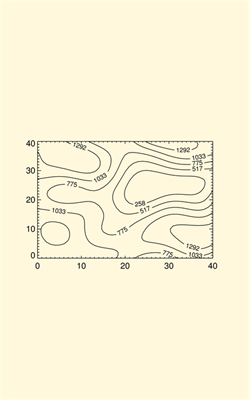# Creating IDL Graphics with Specified Aspect Ratios

QUESTION: How do I create an IDL graphics plot with a specified aspect ratio?ANSWER: Creating an IDL graphic with a specified aspect ratio (the ratio of height to width) requires you to know the size of the graphics output window and how to position an IDL graphic in that window. The size of the graphics window is given by the system variables !D.X_VSIZE and !D.Y_VSIZE in device or pixel coordinates. You must position the IDL graphic in the window with the Position keyword.

I use the IDL program cgAspect to calculate the POSITION coordinates of the graphic in the current IDL graphics window. (The current IDL graphics window is always indicated by the system variable !D.WINDOW.) The cgAspect program works as well for PostScript output as it does for output on the display.

For example, suppose I want to position a contour plot in the current display window and I want the contour plot to have an aspect ratio of 2:3. I do this:

```   data = cgDemoData(2)
plotPosition = Aspect(2.0/3.0)
cgContour, data, Position=plotPosition
```

The aspect ratio of the plot can also be set by using the Aspect keyword of an Coyote Graphics command. Here, for example, is how you can create a square contour plot (i.e., with an aspect ratio of 1).

```   data = cgDemoData(2)
cgContour, data, Aspect=1.0
```

By default, the cgAspectprogram positions the graphic in the current display window with a margin of 0.15 in normalized coordinates units. I can change that if I like with the Margin keyword. For example, suppose I want the plot to fill up most of the window, I can do this:

```   data = cgDemoData(2)
plotPosition = Aspect(2.0/3.0, MARGIN=0.05)
cgContour, data, Position=plotPosition
```

Here is an example of a contour plot with an aspect ratio of 2:3 in a window that has an aspect ratio of 8:5.

```   data = cgDemoData(2)
cgDisplay, Aspect=8.0/5.0
cgContour, data, Aspect=2.0/3.0, Background='cornsilk'
```